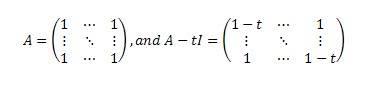# Characteristic polynomial of a matrix

## Homework Statement

Let A be an nxn matrix with real number entries, in which all entries are 1. Find the characteristic polynomial of A.

## Homework Equations

characteristic polynomial: f(t)=det(A-tI), I is identity matrix## The Attempt at a Solution

I've tried to do this by various methods of induction, only to encounter massive blocks along the way. I can't really do anything with this past plug in numbers and hope something works. I know the equation should be (-1)ntn-1(t-n), but I don't know how to prove it. This is from a section of my book on invariant subspaces, is there anything I could do with those?

vela
Staff Emeritus
Homework Helper
I have a suggestion, though it may be misguided. Try finding the null space and image of A. The vectors that span the null space are eigenvectors with eigenvalue 0. From the image, you should be able to identify the remaining eigenvector and its eigenvalue. From that, you can deduce everything except the factor of (-1)n.

Well, one way to do it is to use the formula for determinant

$$det A = \epsilon_{i_1i_2...i_N}A_{i_11}A_{i_22}...A_{i_NN}$$

For your case $A_{ij} = 1 - t\delta_{ij}$

Also useful, will be the fact

$$\sum\limits_{i=1}^N\sum\limits_{j=1}^N \epsilon_{ijklm....} = 0$$